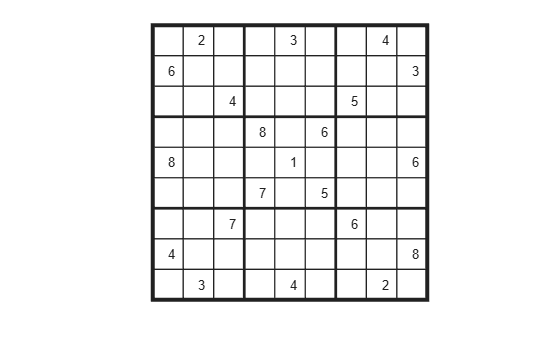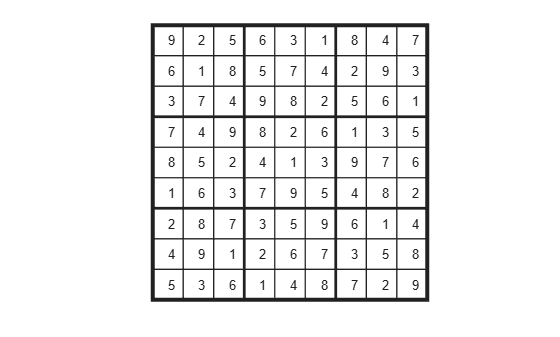Solve Sudoku Puzzles Via Integer Programming: Solver-Based

This example shows how to solve a Sudoku puzzle using binary integer programming. For the problem-based approach, see Solve Sudoku Puzzles Via Integer Programming: Problem-Based.

You probably have seen Sudoku puzzles. A puzzle is to fill a 9-by-9 grid with integers from 1 through 9 so that each integer appears only once in each row, column, and major 3-by-3 square. The grid is partially populated with clues, and your task is to fill in the rest of the grid.

Initial Puzzle

Here is a data matrix B of clues. The first row, B(1,2,2), means row 1, column 2 has a clue 2. The second row, B(1,5,3), means row 1, column 5 has a clue 3. Here is the entire matrix B.

B = [1,2,2;
1,5,3;
1,8,4;
2,1,6;
2,9,3;
3,3,4;
3,7,5;
4,4,8;
4,6,6;
5,1,8;
5,5,1;
5,9,6;
6,4,7;
6,6,5;
7,3,7;
7,7,6;
8,1,4;
8,9,8;
9,2,3;
9,5,4;
9,8,2];

drawSudoku(B) % For the listing of this program, see the end of this example.This puzzle, and an alternative MATLAB® solution technique, was featured in Cleve's Corner in 2009.

There are many approaches to solving Sudoku puzzles manually, as well as many programmatic approaches. This example shows a straightforward approach using binary integer programming.

This approach is particularly simple because you do not give a solution algorithm. Just express the rules of Sudoku, express the clues as constraints on the solution, and then intlinprog produces the solution.

Binary Integer Programming Approach

The key idea is to transform a puzzle from a square 9-by-9 grid to a cubic 9-by-9-by-9 array of binary values (0 or 1). Think of the cubic array as being 9 square grids stacked on top of each other. The top grid, a square layer of the array, has a 1 wherever the solution or clue has a 1. The second layer has a 1 wherever the solution or clue has a 2. The ninth layer has a 1 wherever the solution or clue has a 9.

This formulation is precisely suited for binary integer programming.

The objective function is not needed here, and might as well be 0. The problem is really just to find a feasible solution, meaning one that satisfies all the constraints. However, for tie breaking in the internals of the integer programming solver, giving increased solution speed, use a nonconstant objective function.

Express the Rules for Sudoku as Constraints

Suppose a solution $x$ is represented in a 9-by-9-by-9 binary array. What properties does $x$ have? First, each square in the 2-D grid (i,j) has exactly one value, so there is exactly one nonzero element among the 3-D array entries $x\left(i,j,1\right),...,x\left(i,j,9\right)$. In other words, for every $i$ and $j$,

$\sum _{k=1}^{9}x\left(i,j,k\right)=1.$

Similarly, in each row $i$ of the 2-D grid, there is exactly one value out of each of the digits from 1 to 9. In other words, for each $i$ and $k$,

$\sum _{j=1}^{9}x\left(i,j,k\right)=1.$

And each column $j$ in the 2-D grid has the same property: for each $j$ and $k$,

$\sum _{i=1}^{9}x\left(i,j,k\right)=1.$

The major 3-by-3 grids have a similar constraint. For the grid elements $1\le i\le 3$ and $1\le j\le 3$, and for each $1\le k\le 9$,

$\sum _{i=1}^{3}\sum _{j=1}^{3}x\left(i,j,k\right)=1.$

To represent all nine major grids, just add 3 or 6 to each $i$ and $j$ index:

$\sum _{i=1}^{3}\sum _{j=1}^{3}x\left(i+U,j+V,k\right)=1,$ where $U,V\phantom{\rule{0.5em}{0ex}}ϵ\phantom{\rule{0.5em}{0ex}}\left\{0,3,6\right\}.$

Express Clues

Each initial value (clue) can be expressed as a constraint. Suppose that the $\left(i,j\right)$ clue is $m$ for some $1\le m\le 9$. Then $x\left(i,j,m\right)=1$. The constraint $\sum _{k=1}^{9}x\left(i,j,k\right)=1$ ensures that all other $x\left(i,j,k\right)=0$ for $k\ne m$.

Write the Rules for Sudoku

Although the Sudoku rules are conveniently expressed in terms of a 9-by-9-by-9 solution array x, linear constraints are given in terms of a vector solution matrix x(:). Therefore, when you write a Sudoku program, you have to use constraint matrices derived from 9-by-9-by-9 initial arrays.

Here is one approach to set up Sudoku rules, and also include the clues as constraints. The sudokuEngine file comes with your software.

type sudokuEngine
function [S,eflag] = sudokuEngine(B)
% This function sets up the rules for Sudoku. It reads in the puzzle
% expressed in matrix B, calls intlinprog to solve the puzzle, and returns
% the solution in matrix S.
%
% The matrix B should have 3 columns and at least 17 rows (because a Sudoku
% puzzle needs at least 17 entries to be uniquely solvable). The first two
% elements in each row are the i,j coordinates of a clue, and the third
% element is the value of the clue, an integer from 1 to 9. If B is a
% 9-by-9 matrix, the function first converts it to 3-column form.

%   Copyright 2014 The MathWorks, Inc.

if isequal(size(B),[9,9]) % 9-by-9 clues
% Convert to 81-by-3
[SM,SN] = meshgrid(1:9); % make i,j entries
B = [SN(:),SM(:),B(:)]; % i,j,k rows
% Now delete zero rows
[rrem,~] = find(B(:,3) == 0);
B(rrem,:) = [];
end

if size(B,2) ~= 3 || length(size(B)) > 2
error('The input matrix must be N-by-3 or 9-by-9')
end

if sum([any(B ~= round(B)),any(B < 1),any(B > 9)]) % enforces entries 1-9
error('Entries must be integers from 1 to 9')
end

%% The rules of Sudoku:
N = 9^3; % number of independent variables in x, a 9-by-9-by-9 array
M = 4*9^2; % number of constraints, see the construction of Aeq
Aeq = zeros(M,N); % allocate equality constraint matrix Aeq*x = beq
beq = ones(M,1); % allocate constant vector beq
f = (1:N)'; % the objective can be anything, but having nonconstant f can speed the solver
lb = zeros(9,9,9); % an initial zero array
ub = lb+1; % upper bound array to give binary variables

counter = 1;
for j = 1:9 % one in each row
for k = 1:9
Astuff = lb; % clear Astuff
Astuff(1:end,j,k) = 1; % one row in Aeq*x = beq
Aeq(counter,:) = Astuff(:)'; % put Astuff in a row of Aeq
counter = counter + 1;
end
end

for i = 1:9 % one in each column
for k = 1:9
Astuff = lb;
Astuff(i,1:end,k) = 1;
Aeq(counter,:) = Astuff(:)';
counter = counter + 1;
end
end

for U = 0:3:6 % one in each square
for V = 0:3:6
for k = 1:9
Astuff = lb;
Astuff(U+(1:3),V+(1:3),k) = 1;
Aeq(counter,:) = Astuff(:)';
counter = counter + 1;
end
end
end

for i = 1:9 % one in each depth
for j = 1:9
Astuff = lb;
Astuff(i,j,1:end) = 1;
Aeq(counter,:) = Astuff(:)';
counter = counter + 1;
end
end

%% Put the particular puzzle in the constraints
% Include the initial clues in the |lb| array by setting corresponding
% entries to 1. This forces the solution to have |x(i,j,k) = 1|.

for i = 1:size(B,1)
lb(B(i,1),B(i,2),B(i,3)) = 1;
end

%% Solve the Puzzle
% The Sudoku problem is complete: the rules are represented in the |Aeq|
% and |beq| matrices, and the clues are ones in the |lb| array. Solve the
% problem by calling |intlinprog|. Ensure that the integer program has all
% binary variables by setting the intcon argument to |1:N|, with lower and
% upper bounds of 0 and 1.

intcon = 1:N;

[x,~,eflag] = intlinprog(f,intcon,[],[],Aeq,beq,lb,ub);

%% Convert the Solution to a Usable Form
% To go from the solution x to a Sudoku grid, simply add up the numbers at
% each \$(i,j)\$ entry, multiplied by the depth at which the numbers appear:

if eflag > 0 % good solution
x = reshape(x,9,9,9); % change back to a 9-by-9-by-9 array
x = round(x); % clean up non-integer solutions
y = ones(size(x));
for k = 2:9
y(:,:,k) = k; % multiplier for each depth k
end

S = x.*y; % multiply each entry by its depth
S = sum(S,3); % S is 9-by-9 and holds the solved puzzle
else
S = [];
end

Call the Sudoku Solver

S = sudokuEngine(B); % Solves the puzzle pictured at the start
LP:                Optimal objective value is 29565.000000.

Optimal solution found.

Intlinprog stopped at the root node because the objective value is within a gap
tolerance of the optimal value, options.AbsoluteGapTolerance = 0 (the default
value). The intcon variables are integer within tolerance,
options.IntegerTolerance = 1e-05 (the default value).
drawSudoku(S)You can easily check that the solution is correct.

Function to Draw the Sudoku Puzzle

type drawSudoku
function drawSudoku(B)
% Function for drawing the Sudoku board

%   Copyright 2014 The MathWorks, Inc.

figure;hold on;axis off;axis equal % prepare to draw
rectangle('Position',[0 0 9 9],'LineWidth',3,'Clipping','off') % outside border
rectangle('Position',[3,0,3,9],'LineWidth',2) % heavy vertical lines
rectangle('Position',[0,3,9,3],'LineWidth',2) % heavy horizontal lines
rectangle('Position',[0,1,9,1],'LineWidth',1) % minor horizontal lines
rectangle('Position',[0,4,9,1],'LineWidth',1)
rectangle('Position',[0,7,9,1],'LineWidth',1)
rectangle('Position',[1,0,1,9],'LineWidth',1) % minor vertical lines
rectangle('Position',[4,0,1,9],'LineWidth',1)
rectangle('Position',[7,0,1,9],'LineWidth',1)

% Fill in the clues
%
% The rows of B are of the form (i,j,k) where i is the row counting from
% the top, j is the column, and k is the clue. To place the entries in the
% boxes, j is the horizontal distance, 10-i is the vertical distance, and
% we subtract 0.5 to center the clue in the box.
%
% If B is a 9-by-9 matrix, convert it to 3 columns first

if size(B,2) == 9 % 9 columns
[SM,SN] = meshgrid(1:9); % make i,j entries
B = [SN(:),SM(:),B(:)]; % i,j,k rows
end

for ii = 1:size(B,1)
text(B(ii,2)-0.5,9.5-B(ii,1),num2str(B(ii,3)))
end

hold off

end